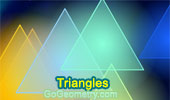v# Triangles: Theorems and Problems 7Geometry Problem 1225
Equilateral Triangle, Circumcircle, Angle Bisector, Congruence.

Geometry Problem 1224
Triangle, Parallel to the Sides.

Geometry Problem 1222
Doubling the Cube, Triangle, 90, 120 degrees, Perpendicular, Congruence.

Geometry Problem 1220
Equilateral Triangles, Midpoints, 60 Degrees, Congruence.

Geometry Problem 1219
Equilateral Triangles, Midpoints, 60 Degrees, Congruence, Rhombus.

Geometry Problem 1218
Triangle, Equilateral Triangles, Midpoints.

Geometry Problem 1217
Triangle, Circle, Excenter, Incenter, Angle Bisector, Cyclic Quadrilateral, Circumcircle, Tangent Line.

Geometry Problem 1215
Circle, Diameter, Chord, Radius, Midpoint, Measurement.

Geometry Problem 1214
Triangle, Three Angle Bisectors, Proportions.

Geometry Problem 1213
Triangle, Circumcircle, Altitudes, Cyclic Quadrilateral, Congruence.

Geometry Problem 1212
Equilateral Triangle, Equilateral Hexagon, Concurrent Lines.

Geometry Problem 1211
Right Triangle, Altitude, Angle Bisector, 45 Degrees.

Geometry Problem 1209
Triangle, Circle, Excircle, Excenter, Circumcircle, Congruence.

Geometry Problem 1208
Triangle, Circle, Excircle, Excenter, Diameter, Perpendicular, 90 Degrees, Equal Areas.

Geometry Problem 1207
Triangle, Circle, Incenter, Circumcenter, Excenter, Circumradius, Perpendicular, 90 Degrees.

Geometry Problem 1206
Circle, Angle Bisector, Secant, Triangle, Circumcircle, Congruence, Area.

Geometry Problem 1205
Triangle, Centroid, Outer and Inner Napoleon Equilateral Triangles.

Geometry Problem 1204
Square, Triangle, Area.

Geometry Problem 1203
Right Triangle, Square, Angle Bisector, Three Congruent Quadrilaterals.

Geometry Problem 1201
Triangle, Interior Perpendicular Bisector, Midpoint, Area.

Geometry Problem 1200
Square, Right Triangle, Perpendicular, 90 Degrees, Equal Areas.

Geometry Problem 1199
Equilateral Triangle, Square, Altitude, Circle, Incircle, Inradius, Metric Relations.

Geometry Problem 1198
Right Triangle, Square, Rectangle, Quadrilateral, Altitude, Equal Areas.

Geometry Problem 1197
Four Squares, Four Triangles, Equal Areas.

Geometry Problem 1196
Parallelogram, Midpoint, Metric Relations.

Geometry Problem 1194
Equilateral Triangle, Perpendicular, Distance, Side, Metric Relations.

Geometry Problem 1193
3-4-5 Right Triangle, Congruent Circles, Tangent, Radius.

Geometry Problem 1192
Trapezoid, Triangle, Diagonal, Midpoint, Area, Parallel.

Geometry Problem 1188
Triangle, Parallelogram, Parallel Lines, Trapezoid, Area.

Go to Page: Previous | 1 | 2 | 3 | 4 | 5 | 6 | 7 | 8 | 9 | 10 | 11 | 12 | 13 | 14 | 15 | 16 | 17 | 18 | 19 | 20 | 21 | 22 | 23 | 24 | 25 | 26 | 27 | 28 | 29 | 30 | 40 | Next

 Home | Search | Geometry | Polygon | Email | By Antonio Gutierrez